Anzeige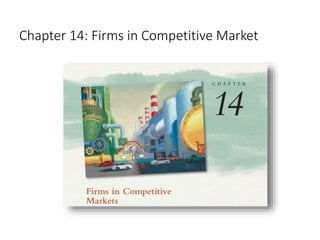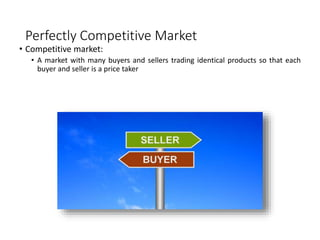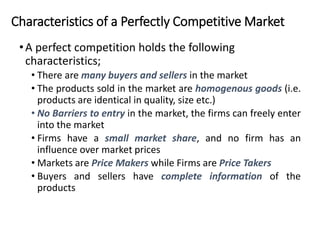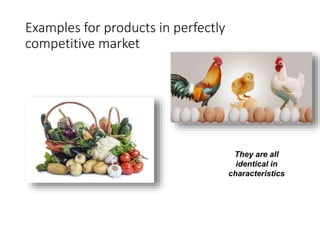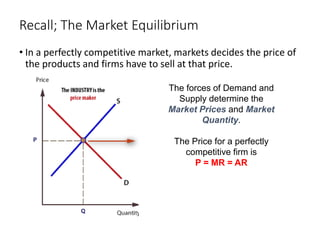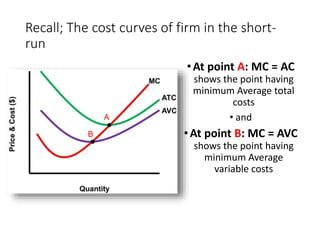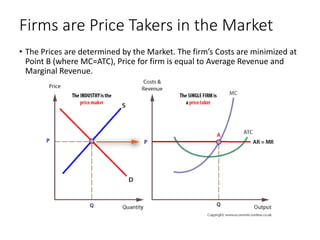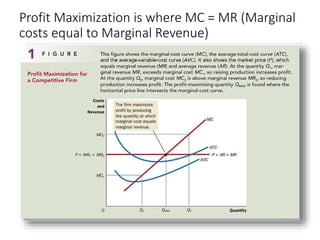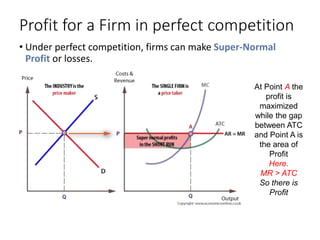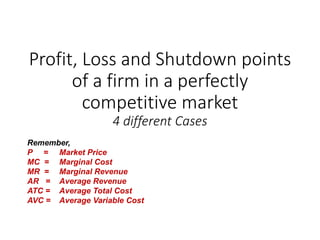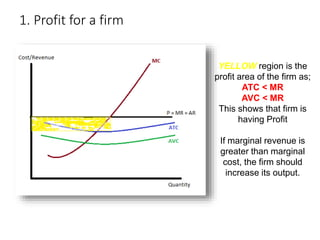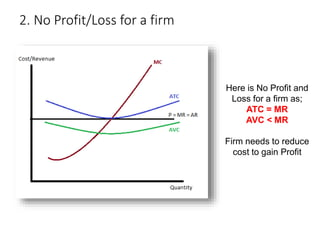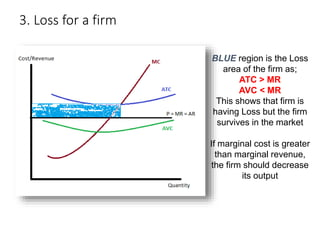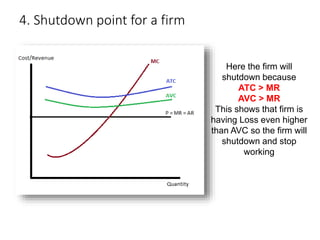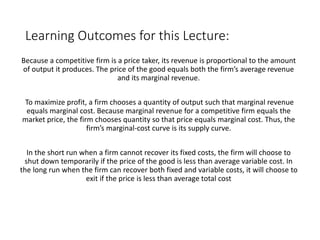1 von 16
Anzeige

### Perfectly Competitive Market.ppt

1. Chapter 14: Firms in Competitive Market
2. Perfectly Competitive Market • Competitive market: • A market with many buyers and sellers trading identical products so that each buyer and seller is a price taker
3. Characteristics of a Perfectly Competitive Market •A perfect competition holds the following characteristics; • There are many buyers and sellers in the market • The products sold in the market are homogenous goods (i.e. products are identical in quality, size etc.) • No Barriers to entry in the market, the firms can freely enter into the market • Firms have a small market share, and no firm has an influence over market prices • Markets are Price Makers while Firms are Price Takers • Buyers and sellers have complete information of the products
4. Examples for products in perfectly competitive market They are all identical in characteristics
5. Recall; The Market Equilibrium • In a perfectly competitive market, markets decides the price of the products and firms have to sell at that price. The forces of Demand and Supply determine the Market Prices and Market Quantity. The Price for a perfectly competitive firm is P = MR = AR
6. Recall; The cost curves of firm in the short- run •At point A: MC = AC shows the point having minimum Average total costs • and •At point B: MC = AVC shows the point having minimum Average variable costs A B
7. Firms are Price Takers in the Market • The Prices are determined by the Market. The firm’s Costs are minimized at Point B (where MC=ATC), Price for firm is equal to Average Revenue and Marginal Revenue.
8. Profit Maximization is where MC = MR (Marginal costs equal to Marginal Revenue)
9. Profit for a Firm in perfect competition • Under perfect competition, firms can make Super-Normal Profit or losses. At Point A the profit is maximized while the gap between ATC and Point A is the area of Profit Here. MR > ATC So there is Profit
10. Profit, Loss and Shutdown points of a firm in a perfectly competitive market 4 different Cases Remember, P = Market Price MC = Marginal Cost MR = Marginal Revenue AR = Average Revenue ATC = Average Total Cost AVC = Average Variable Cost
11. 1. Profit for a firm YELLOW region is the profit area of the firm as; ATC < MR AVC < MR This shows that firm is having Profit If marginal revenue is greater than marginal cost, the firm should increase its output.
12. 2. No Profit/Loss for a firm Here is No Profit and Loss for a firm as; ATC = MR AVC < MR Firm needs to reduce cost to gain Profit
13. 3. Loss for a firm BLUE region is the Loss area of the firm as; ATC > MR AVC < MR This shows that firm is having Loss but the firm survives in the market If marginal cost is greater than marginal revenue, the firm should decrease its output
14. 4. Shutdown point for a firm Here the firm will shutdown because ATC > MR AVC > MR This shows that firm is having Loss even higher than AVC so the firm will shutdown and stop working
15. Learning Outcomes for this Lecture: Because a competitive firm is a price taker, its revenue is proportional to the amount of output it produces. The price of the good equals both the firm’s average revenue and its marginal revenue. To maximize profit, a firm chooses a quantity of output such that marginal revenue equals marginal cost. Because marginal revenue for a competitive firm equals the market price, the firm chooses quantity so that price equals marginal cost. Thus, the firm’s marginal-cost curve is its supply curve. In the short run when a firm cannot recover its fixed costs, the firm will choose to shut down temporarily if the price of the good is less than average variable cost. In the long run when the firm can recover both fixed and variable costs, it will choose to exit if the price is less than average total cost
16. Thank you for Listening

1. r
Anzeige